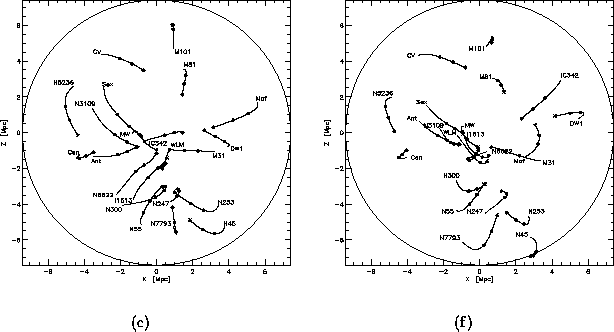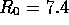Next: Review of the AVP Up: Orbits of Galaxies in Previous: Extended Evaluation of Optimizing

## A Physical Discussion

Mainly due to the poor quality of observations, especially when it comes to mass and distance, the fit of observed to predicted radial velocities of the galaxies in Table 5.4 is at best qualitative. In addition, the multivalued nature of the solutions makes it difficult to pick the solution that best represent the actual galaxy orbits.

The criterion for best fit of the solution of the system to observed line-of-sight velocities is the same as was used when determining the mass of the system in Subsection 5.4.1, namely by considering the radial velocities of the four dwarf galaxies in the LG: NGC 6822, M31, IC 1613, and WLM. The observations of the redshifts of these galaxies are usually accepted to be adequate, and it is therefore fair to assume that any correct solution of the AVP should be able to represent the velocity of these galaxies to within a certain limit of accuracy. P-96 set this limit to 75 km sfor four of the closest dwarf galaxies to the MW in his system; Fornax, Leo I, Leo II, and NGC 6822. Although different galaxies are considered here, the same limit is adopted.

The allowed solution that gave the best fit was located by the Secant method with backtracking. This was a minimum point with an average error of fit for the four galaxies of about 30 km s. The resultant orbits of this solution are presented in Figure 5.4(a), and a comparison between predicted and observed velocities is given in Figure 5.5(a), the former is plotted against the latter. The predicted velocities in this solution are also given in column (8) of Table 5.4.Figure: Orbits for a system of 22 mass tracers. (a): Best fit for the original system with distances given in column (4) of Table 5.4. (b): Best fit for a a system where the distances of the original system have been adjusted to give a better fit than in (a). Adjusted distances is given in column (9) of Table 5.4. The present radius of the circle isMpc.

Figure 5.5(a) clearly shows that even though the fit for the galaxies in the LG is adequate, most of the galaxies in the LN have predicted velocities that are quite different from the observed values. Most erroneous are the velocities for M101 and NGC 45, which are off by as much as about 200-300 km s(for this reason, M101 is not included in Figure 5.5(a)--it having a positive velocity of 570 km s). These rather large offsets can be explained by quite a few factors, the major ones being an incomplete system of mass tracers, a rough mass model, wrong cosmological model, and inaccurate distance observations. Not to be forgotten is the problem with the multitude of solutions given by the AVP. It may very well be that the correct solution has not been detected by the search in the previous subsection.Figure: Predicted velocities plotted against observed values. (a): Best fit for the original system with distances given in column (4) of Table 5.4. (b): Best fit for a system where the distances of the original system has been adjusted to give a better fit than in (a). Adjusted distances given in column (9) of Table 5.4.

In an attempt to better fit the radial velocities given by the model to observations, the distances to the galaxies in the LN can be adjusted, thereby giving an estimate of what the distances are supposed to be according to the AVP. It is important to note that these adjustments of distances are based on the assumptions that the system of galaxies in Table 5.4 correctly represent the mass distribution in the LG and the LN, and that both the mass model and the cosmological model are correct. Neither of these factors are probably correct, but the adjustments of these factors will not be considered here.

The technique of adjusting the distances to get a closer agreement between the model and observations was tried. Some of the galaxies in the LN were given alternative distances and a search for solutions starting from a large number of different initial values was undertaken. Based on the results from the previous section, the Secant method with backtracking was used due to its ability to find a large number of minimum points. The adjusted distances is given in column (9) of Table 5.4, the resultant orbits for the best fit is presented in Figure 5.4(b), and the comparison of predicted and observed velocities is given in Figure 5.5(b).

By comparing the two figures in Figure 5.5, the distance adjustments improved the fit notably, especially for the more distant mass tracers. An even better fit could have been achieved by adjusting the distance to all the mass tracers, but a perfect fit would most probably not be a good representation of the actual distances due to the uncertainty in the mass model and the radial velocities, and the incompleteness of the system. The distance to the galaxies in the LG and the ones closest to the LG were not adjusted due to the relatively high accuracy in the distance estimates, indicated by the use of two decimals in column (4) of Table 5.4. The discrepancy between the observed and predicted velocities for these galaxies may, in addition to the abovementioned factors, be caused by the erroneous results for the more distant galaxies in the system.

Anyhow, these adjusted distances do give an indication of the distances to the more distant galaxies. For example, the adjusted distance to the M101 group is within the range of the distance estimates in literature [][and references therein]Stet, and the Sculptor galaxies are given new distances which are in line with the distance given to the Sculptor group by P-96. The Dw1 galaxy has been given a distance that lies somewhat outside the range presented by Phil, but the observations of this galaxy are very inaccurate, both when it comes to radial velocity and mass, in addition to the distance.

A note on the Antlia galaxy. From observations, there are indications of Antlia being a companion of NGC 3109. The majority of the solutions discovered in this thesis shows that the two galaxies are either closing in on each other for the first time, or they have just passed by. This indicate that the two-galaxy system is not virialized, and that they therefore are not relaxed companions. Although, it will probably be advantageous to treat these two as one mass tracer, reducing the multivalued nature of the solutions. The same argument goes for the Sculptor group galaxies.Next: Review of the AVP Up: Orbits of Galaxies in Previous: Extended Evaluation of Optimizing

Trond Hjorteland
Mon Jul 5 02:59:28 MET DST 1999